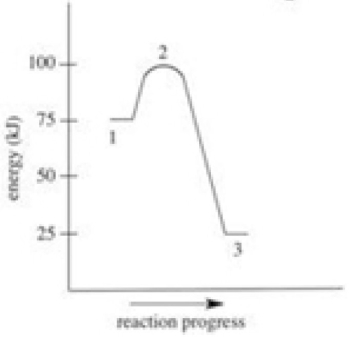Try out our new practice tests completely free!# Quiz 7: Energy,rate and EquilibriumWhich of the following is FALSE about the energy diagram shown?(Multiple Choice)

AWhich of the following statements concerning the reaction below is FALSE? 2HgO(s)→ 2Hg(l)+ O2(g)∆H = 182 kJ(Multiple Choice)

DA carbohydrate sample weighing 0.235 g was found to have a fuel value of 3.84 kJ.What is the fuel value of one gram of this carbohydrate,in nutritional Calories? [1 cal = 4.18 J](Multiple Choice)

EWhich statement concerning energy changes in chemical reactions is FALSE?
(Multiple Choice)The rate of a chemical reaction increases with an increase in concentration of one or more of the reactants.This is best explained by which of the following statements?
(Multiple Choice)The amount of heat necessary to raise 1 gram of a substance by 1 degree Celsius is known as which of the following?
(Multiple Choice)Which of the following is always necessary for a chemical reaction to occur between two reactants,A and B?
(Multiple Choice)What instrument is used for measuring the heat energy absorbed or released in a chemical reaction?
(Multiple Choice)For the reaction shown below,Keq=2×1011.Which of the following statements concerning this system at equilibrium is true? 2CO(g)+ O2(g)⇌ 2CO2(g)
(Multiple Choice)The rate of a chemical reaction increases with an increase in temperature.Which of the following correctly explains why?
(Multiple Choice)What thermodynamic quantity is the ultimate predictor of reaction spontaneity?
(Multiple Choice)Consider the decomposition of calcium carbonate shown below.Which of the following statements correctly describes this reaction as it occurs in a test tube? CaCO3(s)+ 42.5 kcal → CaO(s)+ CO2(g)
(Multiple Choice)A candy sample is completely combusted in a bomb calorimeter.The calorimeter contains 982 g of water,and the measured temperature increase is 2.62°C.What is the fuel value of the candy sample in nutritional Calories? [SHw = 1.00 cal/(g•°C)]
(Multiple Choice)Which statement concerning a reversible reaction at equilibrium is FALSE?
(Multiple Choice)What is the term used to describe the energy barrier the reactants must overcome in order to form products in a chemical reaction?
(Multiple Choice)The formation of rust (Fe2O3)on an exposed piece of metal typically takes several months.Which of the following statements is NOT a reasonable assumption about this chemical reaction? 4Fe(s)+ 3O2(g)→ 2Fe2O3(s)
(Multiple Choice)What is the equilibrium constant expression for the reaction shown below?
(Multiple Choice)Which statement concerning energy changes in chemical reactions is FALSE?
(Multiple Choice)How many small calories are equivalent to one nutritional Calorie?
(Multiple Choice)What is the name and symbol of the thermodynamic quantity that represents the heat absorbed or liberated in a chemical reaction at constant pressure?
(Multiple Choice)## Filters

• Essay(0)
• Multiple Choice(0)